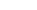•WE PROVIDELINEAR
PROGRAMMING
HW HELP
We can help with
Optimization Problems
Post-Optimal Analysis
and much more
starts at \$25/hour.

# Linear Programming Help

• Linear Programming Examples
• Classical Examples
• Objective Function
• Linear Restrictions
• Graphs

• The Simplex Algorithm
• LP Simplex
• Primal-Dual
• Post Optimal Analysis of Solutions

• LP Software
• Linear Programming graph with Excel
• LINDO API
• Matlab
• GAMS

• Mixed Integer Programming
• Barrier Method
• Integer Programming (Branch and Bound)

• Optimization on Networks
• Transportation Problem
• PERT
• Shortest Path

## Claim Your Free Solved Stats Problems!

* indicates required
Email Format

## Our Specialties

#### Optimization Problems

Modeling an optimization problem can be cumbersome: Find the decision variables, the objective function, the restrictions. Then we need to put the restriction on a form that is suitable for a linear programming setting, compute corner points, graph the feasible region, etc, etc. There is a lot do, and we can help you with that!

#### Simplex

The Simplex method is the classical algorithm used to solve a linear programming problem. It involves using a table and conducting matrix operations in order to add and remove vectors from the basis, until finding the optimal corner point. Our experts have the right expertise to help you with any Simplex problem

#### Excel Solution to LP Problems

Most typically, the linear programming questions involve setting up an Excel spreadsheet and using its Solver's facilities. We can help you with that too.

#### Other Optimization Software

Our experts can help you with other specialized software, such as GAMS, LINDO, Matlab or any other specific software for optimization.